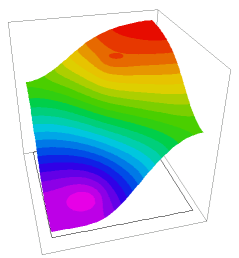﻿ Sample Problems > Usage > 3D_domains > twoz_export

# twoz_export

Navigation:  Sample Problems > Usage > 3D_domains >

# twoz_export{ TWOZ_EXPORT.PDE

This script uses plate-bending equations to generate a surface that

passes through the waist of two spheres of differing Z-coordinates.

The surface is exported with TRANSFER and read into 3D problem

TWOZ_IMPORT.PDE as the layer-dividing surface.

(See "Samples | Applications | Stress | Fixed_Plate.pde" for notes on

plate-bending equations.)

 } title 'Generating extrusion surfaces' variables    U,V definitions    box = 1 { bounding box size }   { read sphere specs from file, to guarantee      the same values as later including script }    #include "sphere_spec.inc"   ! penalty factor to force boundary compliance    big = 1e6    ztable = U equations    U:  del2(U) = V    V:  del2(V) = 0 boundaries   Region 1 { The bounding box }     start(-box,-box)line to (box,-box) to (box,box) to (-box,box) to close

Region 2   { sphere 1 }

ztable = Z1           { force a clean table value inside sphere }

start  (x1+1.01*R1,y1)

mesh_spacing = R1/5   { force a dense mesh on the sphere }

arc(center=x1,y1) angle=360

Region 3     { sphere 2 }

ztable = Z2

start  (x2+1.01*R2,y2)

mesh_spacing = R2/5   { force a dense mesh on the sphere }

arc(center=x2,y2) angle=360

plots

elevation(U) from(-box,-box) to (box,box)

elevation(ztable) from(-box,-box) to (box,box)

contour(U)

surface(U)

contour(ztable) zoom(x1-1.3*R1, y1-1.3*R1, 2.6*R1,2.6*R1)

contour(ztable) zoom(x2-1.3*R2, y2-1.3*R2, 2.6*R2,2.6*R2)

transfer(ztable) file = "two_sphere.xfr"

end